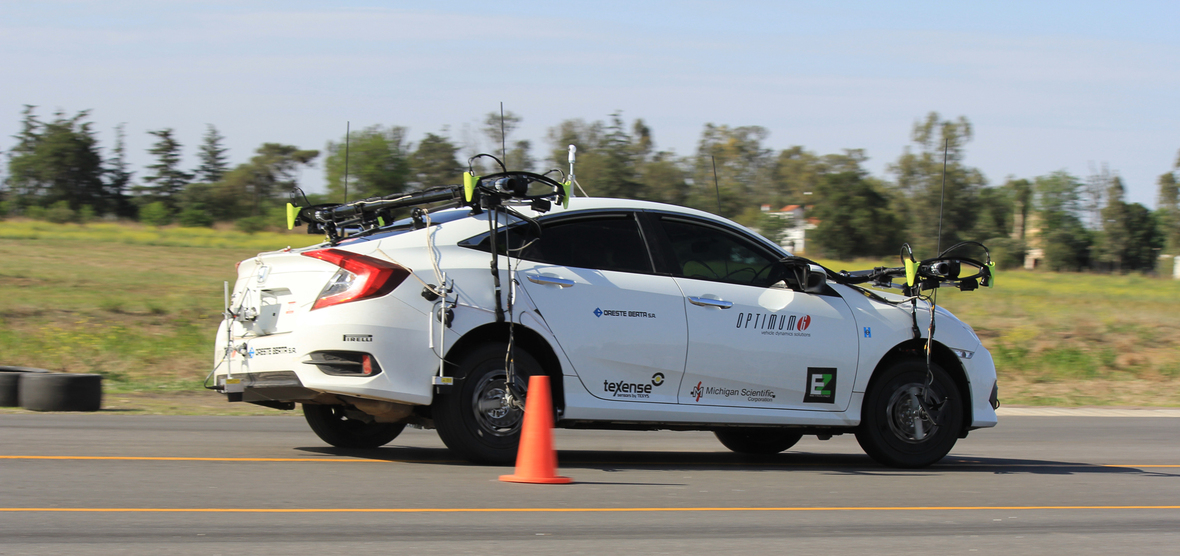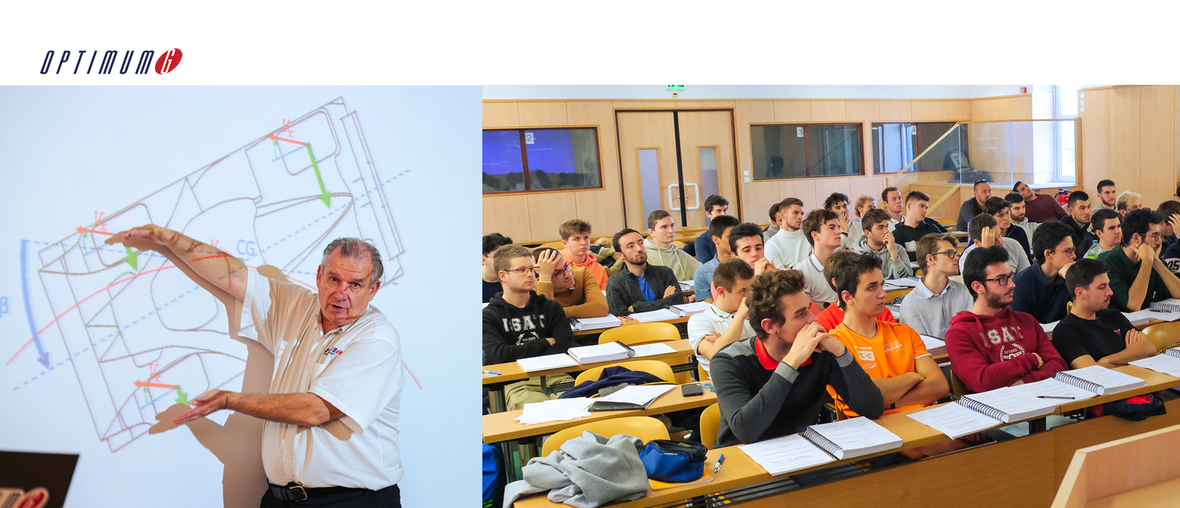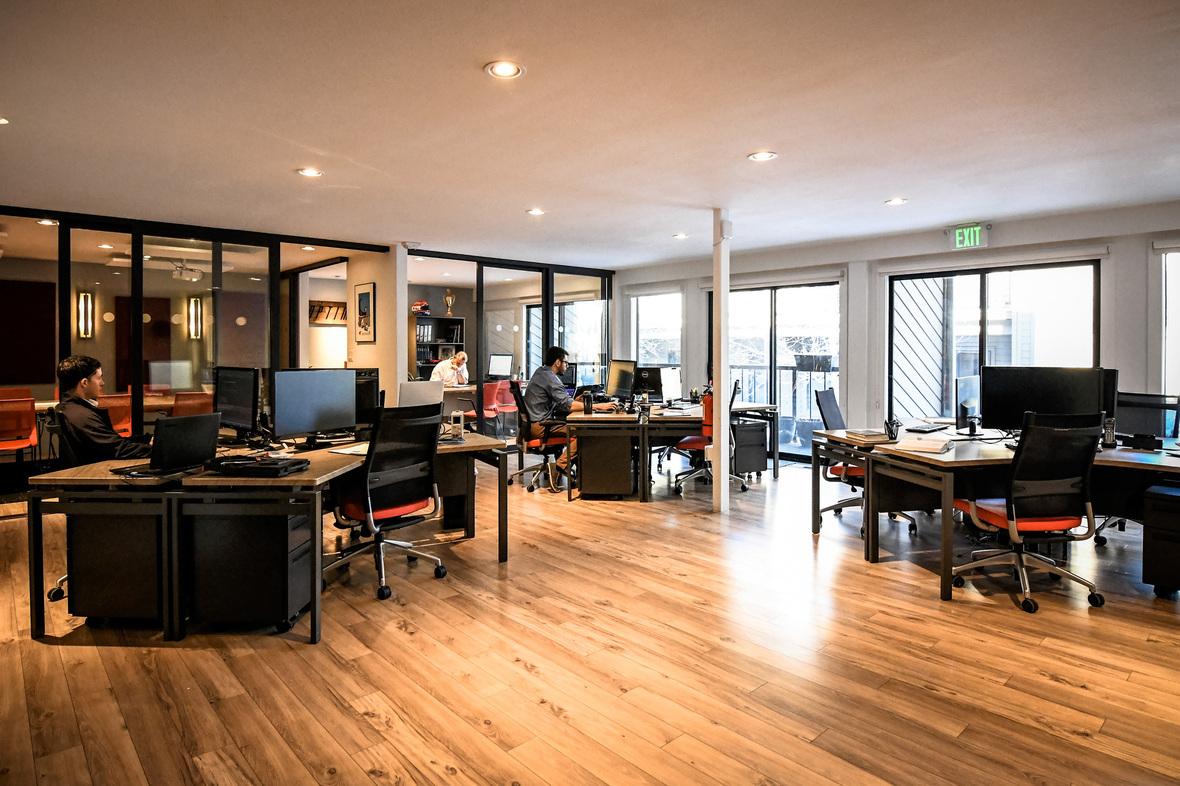Like   Tweet   in

## OptimumG, the essential binding agent in automotive industry and motorsports

 /* styles */ Press Release - February 2022
 table div table+table+table+table+table div table td,table.module-4{width:100%;padding:0}table div table+table+table+table+table div table{width:100%;float:none;margin-left:auto;margin-right:auto;padding:0}table div table+table+table+table+table div table a{border:0 none;text-decoration:none}table div table+table+table+table+table div table img{width:100%!important;border:0 none;text-decoration:none}/* styles */

# We met Claude Rouelle, founder of the company OptimumG.

## Question: Who are you Claude Rouelle?

 table div table+table+table+table+table+table+table+table+table div table,table.module-8{width:50.38%;float:left;padding:0}table div table+table+table+table+table+table+table+table+table div table a{border:0 none;text-decoration:none}table div table+table+table+table+table+table+table+table+table div table img{width:100%!important;border:0 none;text-decoration:none}table div table+table+table+table+table+table+table+table+table div table td{width:100%;padding:0 20px 20px 0}/* styles */ Claude ROUELLE : I am a Belgian citizen. I studied engineering in Liège, where I had the chance to benefit from the university’s social funding. My final project was the building of a racecar, but only one of my professors supported me and the idea. My objective was to design one that I could build myself, but of course, it would have been very expensive to build a Formula One. So, I decided to go for the Formula Ford, a series very much looked at here in Belgium at that time. Even though the school director was not too keen on my project, I built an aerodynamic wind tunnel and a Formula Ford. After a dynamic presentation in front a professional board of examiners, I got a 19/20 grade… The director came to apologize and introduced me to a journalist. After she published her article, one of the most important companies in Belgium announced that I was the recipient of an innovation award. That money enabled me to build my first car, and that's how everything started in 1979. I was 23 years old, and I had become a racecar manufacturer: the Formula Ford was named HIRONDELLE. Even though our race budget was much lower than those of factory teams, we made it to 7th place in the 1980 European Championship.

## Q : You are from Belgium and today you live in the USA. How did you come from one side of the Atlantic to the other?

CR : Yes it’s true I have been living in the United States of America for the past 29 years. But before I ended up in the USA, I spent time in different countries and companies. While I was involved in my first company, Hirondelle, I had the opportunity to work at the Spa 24 Hours in 1983 with Luigi Team and their Alfa Romeo GTV6 and it worked out very well. This was the beginning of a new chapter in my life. I sold my company and spent three years as race engineer with Luigi Racing, before I started with Volvo RAS factory team to end up in 1988/1989 working in F3 with French Company Oreca in the South of France. I also spend some time in F3000 in Japan and Europe, in Formula One with AGS F1, and went to Japan with Reynard for three years. After all that, I came to the USA and worked five full seasons in Indycar before I created OptimumG.

 table div table+table+table+table+table+table+table+table+table+table+table+table div table{width:100%;padding:0}table div table+table+table+table+table+table+table+table+table+table+table+table div table table{padding:0;float:left!important;width:51.132%!important}table div table+table+table+table+table+table+table+table+table+table+table+table div table table+table td,table div table+table+table+table+table+table+table+table+table+table+table+table div table td{padding-left:0;padding-right:0}table div table+table+table+table+table+table+table+table+table+table+table+table div table table td{padding-left:0;padding-right:20px}table div table+table+table+table+table+table+table+table+table+table+table+table div table table+table{float:left!important;width:48.868%!important}/* styles */

## Q : What is OptimumG and what are the different characteristics of the company?

CR : I created OptimumG in 1997, an engineering company, which supplies three different solutions to teams as well as to car- and tire- manufacturers. We supply SOFTWARE solutions, SEMINARS to teach automotive dynamics and CONSULTING support for the teams and manufacturers with R&D and race engineers.At OptimumG, simulations are validated on track with the necessary instruments
 /* styles */ The major objective of OPTIMUMG is obviously to optimize: optimize car set-ups in order for a driver to exploit tires, aerodynamics, brakes, and suspensions… in the best possible way. In order to optimize, we have developed specific software: OptimumTire to model and exploit the tires; OptimumKinematics to simulate and optimize suspension set-ups; OptimumDynamics to study vehicle dynamics and finally OptimumLap to simulate the best lap configuration depending on set-ups.
 table div table+table+table+table+table+table+table+table+table+table+table+table+table+table+table+table+table div table{width:100%;padding:0}table div table+table+table+table+table+table+table+table+table+table+table+table+table+table+table+table+table div table table{padding:0;float:left!important;width:52.641%!important}table div table+table+table+table+table+table+table+table+table+table+table+table+table+table+table+table+table div table table+table td,table div table+table+table+table+table+table+table+table+table+table+table+table+table+table+table+table+table div table td{padding-left:0;padding-right:0}table div table+table+table+table+table+table+table+table+table+table+table+table+table+table+table+table+table div table table td{padding-left:0;padding-right:20px}table div table+table+table+table+table+table+table+table+table+table+table+table+table+table+table+table+table div table table+table{float:left!important;width:47.359%!important}/* styles */
 /* styles */ When engineers need to use these software solutions, they must take a seminar in order to be efficient. This is how we have developed the SEMINAR part of our business. It has become a very important part of OptimumG. Since I created OptimumG, I presented over 400 seminars within companies or not, an average of 20 a year. I’ve taught 14,700 people in 34 different countries. I’ve had seminars with up to 153 participants in India and 269 in Shanghai. Once I had only one student, when Gilles Simon, Ferrari engine technical director asked me to teach a seminar for himself. I love sharing my knowledge. The third OptimumG activity is the CONSULTING branch. We have experienced engineers, who know our products better than anyone does, and they can use our products in different disciplines from endurance racing to single-seater or rallying.Claude Rouelle in front of the class at a seminar in Nevers, France - November 2021

## Q : You create, you produce and you share your knowledge. Don’t you think that you can lose it once you have placed engineers in teams, sold the software and shared your full knowledge?

CR : « Knowledge shared is knowledge squared ».
This is my leitmotiv. Usually, in motorsports as well as in automotive industry, consultants come with a solution but don’t explain how and why.

OptimumG is the opposite, we started with seminars, thus it has always been important to share the knowledge that comes with the software. It works very well and satisfies our clients.

Of course, there’s the risk of a client saying: “Thanks, but now we have all that we need, and we don’t need you anymore.” However, in the past 25 years, nine times out of ten, the client calls us back to support him when he goes to a new category or discipline. When there is new challenge, they want us at their side.

OptimumG works on the vehicle's dynamic between the tire and the bodywork. Of course, it is impossible to find the perfect car set-up if you don’t understand the way the tires work. Whether for racecars and their teams, or for car manufacturers, OptimumG helps to understand the connections which will validate the best set-ups and the best tire characteristics for a team, a car manufacturer or a driver.

In addition, OptimumG can assist a team or a constructor in suspension design, which again will help find the best set-up for an optimal exploitation. OptimumG also organizes objective tire/car- testing for clients such as Goodyear and Hyundai.

 table div table+table+table+table+table+table+table+table+table+table+table+table+table+table+table+table+table+table+table+table+table div table td,table.module-20{width:100%;padding:0}table div table+table+table+table+table+table+table+table+table+table+table+table+table+table+table+table+table+table+table+table+table div table{width:100%;float:none;margin-left:auto;margin-right:auto;padding:0}table div table+table+table+table+table+table+table+table+table+table+table+table+table+table+table+table+table+table+table+table+table div table a{border:0 none;text-decoration:none}table div table+table+table+table+table+table+table+table+table+table+table+table+table+table+table+table+table+table+table+table+table div table img{width:100%!important;border:0 none;text-decoration:none}/* styles */

## Q : So within the different solutions that OptimumG offers, could we say that if a team has an urgent need of a race engineer, he could call you?

CR: It’s not the way we typically work. Nevertheless, teams with an occasional need are welcome to ask, we will offer more than a replacement.

As of today, all the teams we have presented our services to, had the possibility to evaluate the quality of consultation for one full week, and not just only one short day. And every time, the engineer has ended up on the team for the entire season. There is a big difference between CONSULTING and sub-contracting.

## Q : How come we never see OptimumG flags or shirts on racetracks?

CR : OptimumG engineers that are involved with a team are totally integrated. They are fully part of the team and dressed like the team. So, you don’t see them, but they are everywhere. For example, since 2008 OptimumG engineers have worked with Dunlop and now with Goodyear staff. We also have OptimumG engineers in Supercars in Australia with Ford Performance Racing and 888, in Stockcar in Brazil, TC2000 with Peugeot in Argentina, RLL in IMSA and Indycars… We are also present at the Formula One and World Rally Championships, but we won’t say anymore as our privacy agreements are strict.

## Q : There must be a lot of you to do all this? Where is the company based?

CR : OptimumG consists of eleven people full time. We are super-efficient, and we have great team coordination and communication. Team spirit is very important and that is what makes me come to the office every morning with a big smile.

Our office is near Denver Colorado in the USA. It’s very well situated. From here we can fly to London, Munich, Paris or Tokyo. At OptimumG we all love sports and mountains, and here we have it all. It’s perfect.OptimumG offices, Centennial, CO, USA

## Q : Do you have any opposition?

CR : Concerning our SEMINAR, we don’t really have any rivals, not at this level of professionalism. That will happen someday, and that’s exactly why we never stop. Constant improvement in quality and quantity for unique clients is what drives us, and this is also what makes us stronger together.

Concerning the SOFTWARE and CONSULTING businesses, we do have competitors. However, the solutions we have developed to make the right simulation and the full validation of the client’s needs, are part of our strengths. And this strength sometimes gives us the chance to choose our clients.

We want to face new challenges, to take our client to victory and to be able to explain why he has won. In fact, as Hugues de Chaunac, Oreca founder, said at the time I was working with him: “If you don’t know why you win, you will not understand why you lose”

## Q : Where does the name OptimumG come from?

CR: The G Force, is the measure unit for acceleration. OptimumG’s specific jobs are all linked to the optimization of the combination of this G Force in the vehicle dynamics in lateral, longitudinal as well as vertical directions. Our objective: optimize and harmonize to reach performance! So, it was natural to associate these concepts in the company’s name and thus to call it OptimumG.

 table div table+table+table+table+table+table+table+table+table+table+table+table+table+table+table+table+table+table+table+table+table+table+table+table+table+table+table+table div table td,table.module-27{width:100%;padding:0}table div table+table+table+table+table+table+table+table+table+table+table+table+table+table+table+table+table+table+table+table+table+table+table+table+table+table+table+table div table{width:100%;float:none;margin-left:auto;margin-right:auto;padding:0}table div table+table+table+table+table+table+table+table+table+table+table+table+table+table+table+table+table+table+table+table+table+table+table+table+table+table+table+table div table a{border:0 none;text-decoration:none}table div table+table+table+table+table+table+table+table+table+table+table+table+table+table+table+table+table+table+table+table+table+table+table+table+table+table+table+table div table img{width:100%!important;border:0 none;text-decoration:none}/* styles */
 table div table+table+table+table+table+table+table+table+table+table+table+table+table+table+table+table+table+table+table+table+table+table+table+table+table+table+table+table+table div table{width:100%;padding:0}table div table+table+table+table+table+table+table+table+table+table+table+table+table+table+table+table+table+table+table+table+table+table+table+table+table+table+table+table+table div table img{width:96.23%;padding:0;float:none}table div table+table+table+table+table+table+table+table+table+table+table+table+table+table+table+table+table+table+table+table+table+table+table+table+table+table+table+table+table div table td{width:100%;padding:0 1.88% 18px}/* styles *//* styles */ Copyrights Photos : OptimumG; Claude Rouelle; Daniel Delien; Eric Fabre
 table div table+table+table+table+table+table+table+table+table+table+table+table+table+table+table+table+table+table+table+table+table+table+table+table+table+table+table+table+table+table+table div table{width:100%;padding:0}table div table+table+table+table+table+table+table+table+table+table+table+table+table+table+table+table+table+table+table+table+table+table+table+table+table+table+table+table+table+table+table div table img{width:96.23%;padding:0;float:none}table div table+table+table+table+table+table+table+table+table+table+table+table+table+table+table+table+table+table+table+table+table+table+table+table+table+table+table+table+table+table+table div table td{width:100%;padding:0 1.88% 18px}/* styles */# Stay in Touch :

## Tel : + 1 720 427 3584

 table div table+table+table+table+table+table+table+table+table+table+table+table+table+table+table+table+table+table+table+table+table+table+table+table+table+table+table+table+table+table+table+table+table div table{width:100%;padding:0}table div table+table+table+table+table+table+table+table+table+table+table+table+table+table+table+table+table+table+table+table+table+table+table+table+table+table+table+table+table+table+table+table+table div table table{padding:0;float:left!important;width:30.12%!important}table div table+table+table+table+table+table+table+table+table+table+table+table+table+table+table+table+table+table+table+table+table+table+table+table+table+table+table+table+table+table+table+table+table div table td{padding-left:99px;padding-right:99px}table div table+table+table+table+table+table+table+table+table+table+table+table+table+table+table+table+table+table+table+table+table+table+table+table+table+table+table+table+table+table+table+table+table div table table td,table div table+table+table+table+table+table+table+table+table+table+table+table+table+table+table+table+table+table+table+table+table+table+table+table+table+table+table+table+table+table+table+table+table div table table+table td{padding-left:0;padding-right:20px}table div table+table+table+table+table+table+table+table+table+table+table+table+table+table+table+table+table+table+table+table+table+table+table+table+table+table+table+table+table+table+table+table+table div table table+table{float:left!important;width:35.843%!important}table div table+table+table+table+table+table+table+table+table+table+table+table+table+table+table+table+table+table+table+table+table+table+table+table+table+table+table+table+table+table+table+table+table div table table+table+table{float:left!important;width:34.03699999999999%!important}table div table+table+table+table+table+table+table+table+table+table+table+table+table+table+table+table+table+table+table+table+table+table+table+table+table+table+table+table+table+table+table+table+table div table table+table+table td{padding-left:0;padding-right:0}/* styles */
 table div table+table+table+table+table+table+table+table+table+table+table+table+table+table+table+table+table+table+table+table+table+table+table+table+table+table+table+table+table+table+table+table+table+table div table{width:100%;padding:0}table div table+table+table+table+table+table+table+table+table+table+table+table+table+table+table+table+table+table+table+table+table+table+table+table+table+table+table+table+table+table+table+table+table+table div table img{width:96.23%;padding:0;float:none}table div table+table+table+table+table+table+table+table+table+table+table+table+table+table+table+table+table+table+table+table+table+table+table+table+table+table+table+table+table+table+table+table+table+table div table td{width:100%;padding:0 1.88% 18px}/* styles */table div table+table+table+table+table+table+table+table+table+table+table+table+table+table+table+table+table+table+table+table+table+table+table+table+table+table+table+table+table+table+table+table+table+table+table div table{width:100%;padding:0}table div table+table+table+table+table+table+table+table+table+table+table+table+table+table+table+table+table+table+table+table+table+table+table+table+table+table+table+table+table+table+table+table+table+table+table div table img{width:96.23%;padding:0;float:none}table div table+table+table+table+table+table+table+table+table+table+table+table+table+table+table+table+table+table+table+table+table+table+table+table+table+table+table+table+table+table+table+table+table+table+table div table td{width:100%;padding:0 1.88% 18px}/* styles *//* styles */ Contact Media : Mapidu Media Marie-Pierre Dupasquier Damagnez